# How to get an end of month date in Excel?

End of month date means that end date of a provided month. For example if the provided data is “04-apr-2023”, then the last date of month will be “31-apr-2023”. This article focusses on using the predefined method to generate formula to obtain the last month date. Second example of the article is based on the use of “VBA code”. By using the VBA code, user is trained to write a function definition, and after that by calling the function user can easily generate the end date of month in excel.

## Example 1: To get the end of month date in excel by using the formula:

### Step 1:

To understand the method to obtain end of month date by using the formula method. Consider the below given sample worksheet: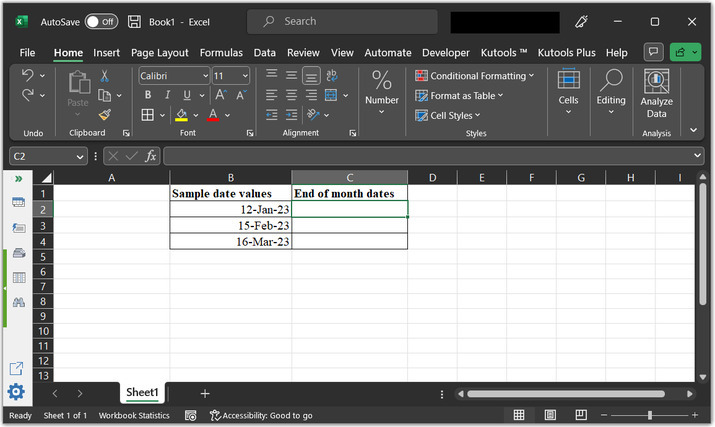### Step 2:

Go to the C2 cell, and type formula “=DATE(YEAR(B2),MONTH(B2)+1,0)”.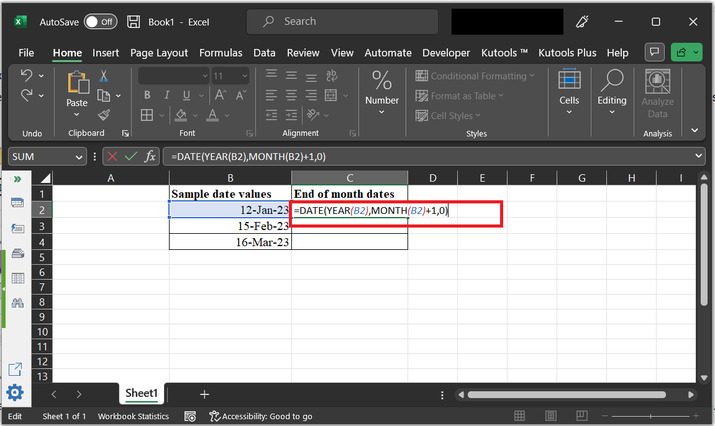### Explanation of above formula

• The DATE() function is a built-in function in Microsoft Excel. It returns a serial number that represents a particular date. The syntax for the date function is provided below:

DATE(year, month, day)

"YEAR(B2)" extracts the year value from the date in cell B2.

"MONTH(B2)+1" adds 1 to the month value of the date in cell B2.

"0" represents the day value. By setting it to 0. Here, user is simply asking Excel to return the day value of the last day of the previous month.

The resulting values from the above steps are used as arguments for the DATE function.

### Step 3:

The above formula will simply calculate a date from the year, month, and day values. To understand the obtained result, consider the below given output.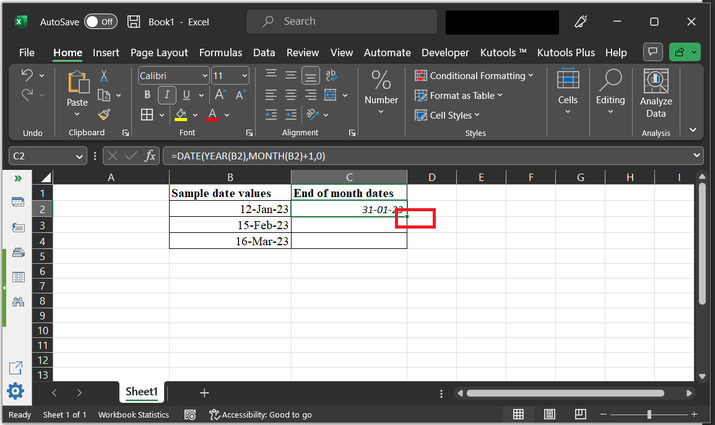### Step 4:

After that click on the bottom of the “C2” cell, and drag results to the bottom of the cell. to copy same formula, to rest of the cells.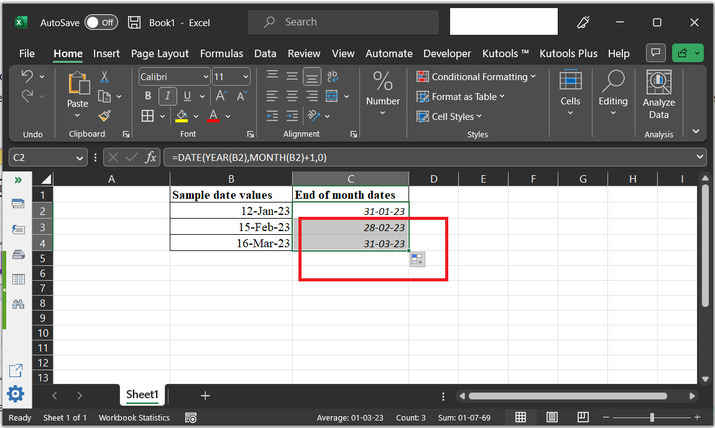## Example 2: To get the end of month date in excel by using the VBA code:

### Step 1:

To understand the example properly, consider the below provided sample worksheet: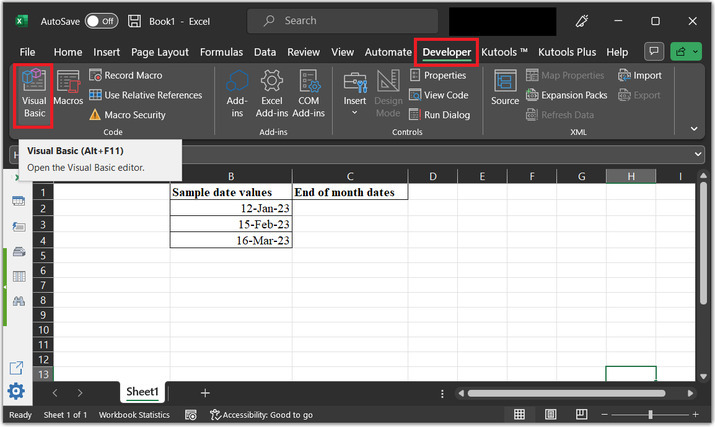### Step 2:

To use the VBA code, user need to open the VBA editor. Go to the “Developer” tab and then under the “code” section, click on “Visual Basic” option. Consider below given image for proper reference:### Step 3:

This will open the “Microsoft Visual Basics or applications” dialog box, as shown below: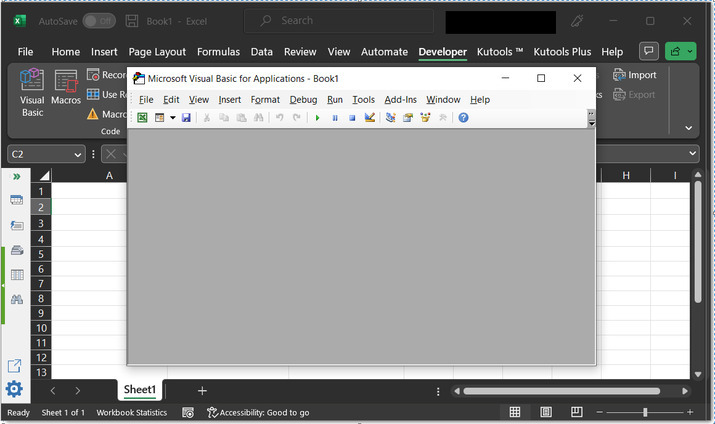### Step 4:

After that in the “Microsoft Visual Basics or applications” dialog box. Go to the “Insert tab” and then choose “Module” option. As depicted below: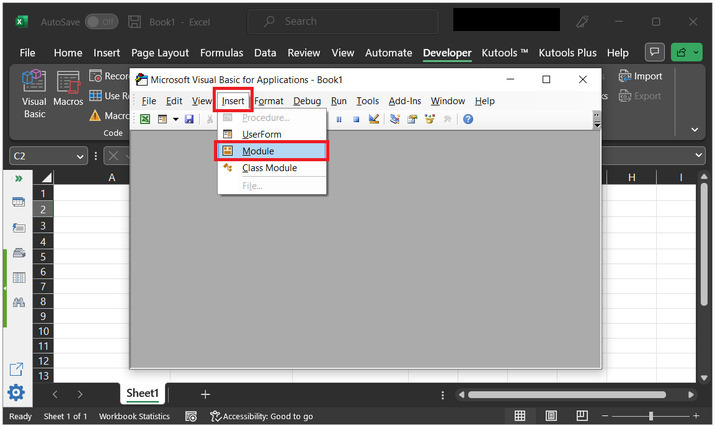### Step 5:

This will open an empty code area. Type the below given code to the empty code area:

' define function name
Function Last_Day_Of_Month(Optional date_var As Date = 0) As Date
' if expression block
If date_var = 0 Then date_var = VBA.Date
' intializing contructor to evaluate last date of
' provided month
Last_Day_Of_Month = VBA.DateSerial(VBA.Year(date_var), VBA.Month(date_var) + 1, 0)
' ending the function module
End Function


### Code snapshot for reference: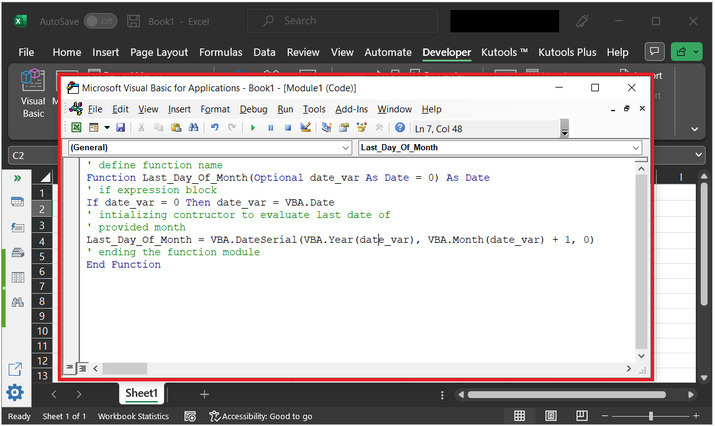### Step 7:

After that come back to the excel sheet and click on the required location. For this case, the considered cell is “C2”. After that type “=Last_Day_Of_Month(B2)” to the cell. Finally, press “Enter” key.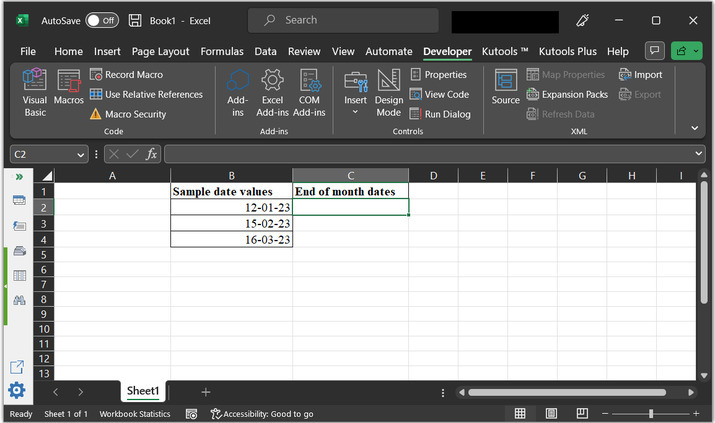### Step 8:

This will type the last date of month to the “C2” column. Consider the below provided snapshot: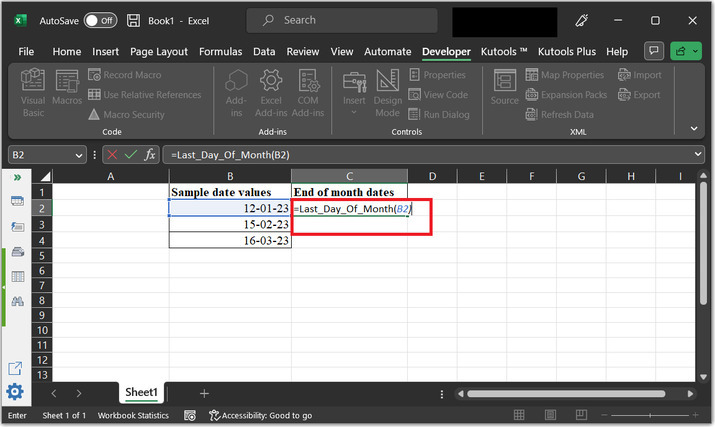### Step 9:

The obtained results are displayed below: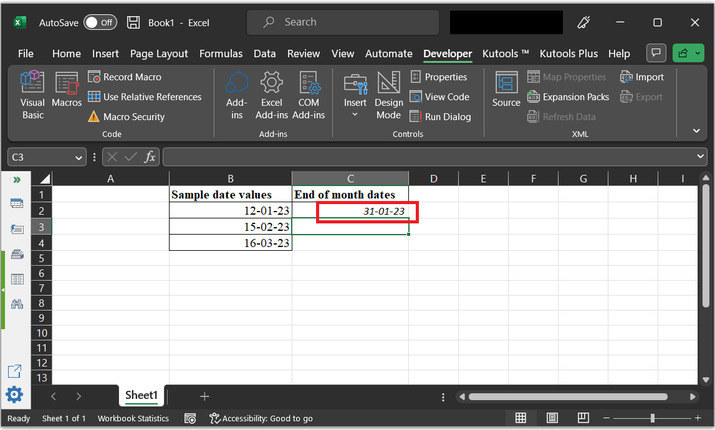### Step 10:

Finally, drag the cell to 4th column, and this will display results as shown below: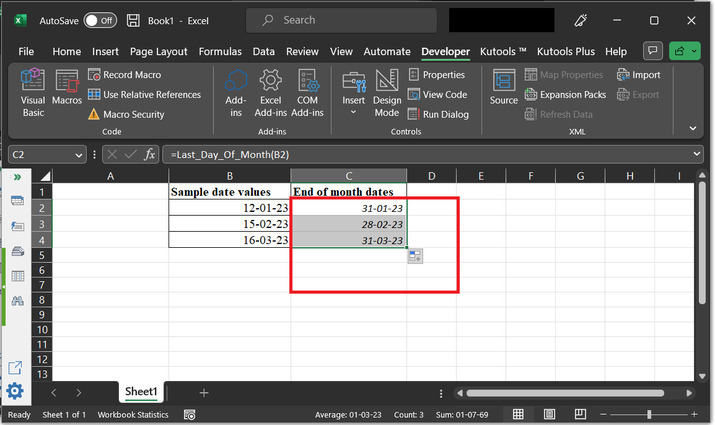## Conclusion:

This article help learner to understand the way to obtain the end date of the month by using the predefined methods, and VBA code. Both the provided methods are efficient and can produce accurate results if steps are followed correctly.

Updated on: 17-Apr-2023

649 Views